# Volume - 6th grade (11y) - math problems

#### Number of problems found: 188

• Volume per timeHow long does fill take for a pump with a volume flow of 200 l per minute fill a cube-shaped tank up to 75% of its height if the length of the cube edge is 4 m?
• Sand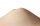How much m ^ 3 of sand can be loaded on a car with a load capacity of 5 t? The sand density is 1600 kg/m ^ 3 .
• The woodenThe wooden block measures 12 cm, 24 cm, and 30 cm. Peter wants to cut it into several identical cubes. At least how many cubes can he get?
• Into boxHow many cubes with an edge of 2.5 cm fit into a box measuring 11.6 cm; 8.9 cm and 13.75 cm?
• Cargo spaceThe cargo space of the truck has the dimensions a = 4.2 m, b = 1.9 m, c = 8.1 dm. Calculate its volume.
• Zhiwei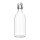Zhiwei wants to divide 19 litres of water equally into 6 pots. Find the volume of water in each pot?
• AngieAngie poured 4 cartons of milk, each with 2.4 litres, into a mixing bowl. How many litres of milk were there altogether?
• AquariumHow many liters of water can fit in an aquarium measuring 30, 15 and 20 cm?
• Ice floe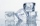What is the volume of an ice floe weighing 326 kg? The density of ice is only 920 kg/m3.
• The bodyThe body has dimensions of 2m 2dm and 10 cm. It weighs 28 kg. What is its density?
• Brick wallWhat is the weight of a solid brick wall that is 30 cm wide, 4 m long and 2 m high? The density of the brick is 1500 kg per cubic meter.
• Thousand ballsWe have to create a thousand balls from a sphere with a diameter of 1 m. What will be their radius?
• Long drive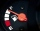Calculate how much it costs to drive 150,000 km with an average consumption of 7 liters per 100 km and a fuel price of 1.2 € / l.
• Mixing paint with waterMr. Adamek will paint. The purchased paint is diluted with water in a ratio of 1: 1.5. a) how many parts of water will add to 1 part of the paint b) how many liters of water the mission adds to 2 liters of paint
• Cylinder containerThe cylindrical container with a diameter of 1.8 m contains 2,000 liters of water. How high does the water reach?
• Empty barrel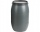In the backyard is an empty barrel with a volume of 2 hl. How many liters are missing at the edge, when 53 liters flowed into it for 1 day and 68 liters of water for 2 days?
• Aquarium heightHow high does the water in the aquarium reach, if there are 36 liters of water in it? The length of the aquarium is 60 cm and the width is 4 dm.
• Cuboid to cubeA cuboid with dimensions of 9 cm, 6 cm, and 4 cm has the same volume as a cube. Calculate the surface of this cube.
• Surface and volumeFind the surface and volume of a cuboid whose dimensions are 1 m, 50 cm, and 6 dm.
• Wooden box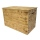The block-shaped box was placed on the ground, leaving a rectangular print with dimensions of 3 m and 2 m. When flipped over to another wall, a print with dimensions of 0.5 m and 3 m remained in the sand. What is the volume of the wooden box?

Do you have an interesting mathematical word problem that you can't solve it? Submit a math problem, and we can try to solve it.

We will send a solution to your e-mail address. Solved examples are also published here. Please enter the e-mail correctly and check whether you don't have a full mailbox.

Please do not submit problems from current active competitions such as Mathematical Olympiad, correspondence seminars etc...